# Practice Test: Time & Work- 2

## 15 Questions MCQ Test IBPS PO Prelims- Study Material, Online Tests, Previous Year | Practice Test: Time & Work- 2

Description
Attempt Practice Test: Time & Work- 2 | 15 questions in 30 minutes | Mock test for Banking Exams preparation | Free important questions MCQ to study IBPS PO Prelims- Study Material, Online Tests, Previous Year for Banking Exams Exam | Download free PDF with solutions
QUESTION: 1

### Mayank can do 50% more work than Shishu in the same time. Shishu alone can do a piece of work in 30 hours. Shishu starts working and he had already worked for 12 hours when Mayank joins him. How many hours should Shishu and Mayank work together to complete the remaining work?

Solution: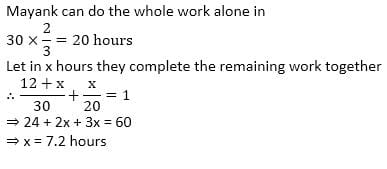QUESTION: 2

### A can do a piece of work in 90 days, B in 40 days and C in 12 days. They work for a day each in turn, i.e., first day A does it alone, second day B does it alone and 3rd day C does it alone. After that the cycle is repeated till the work is finished. They get Rs 240 for this job. If the wages are divided in proportion to the work each had done. Find the amount A will get?

Solution: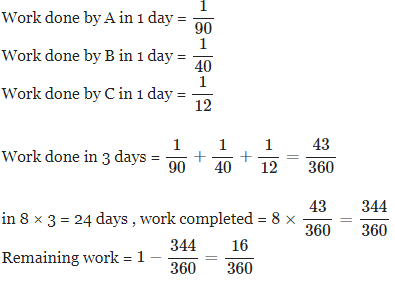.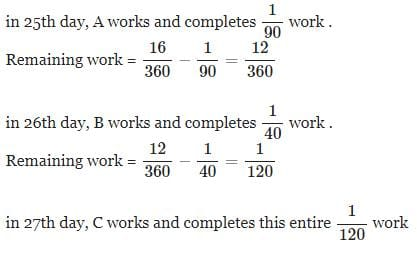QUESTION: 3

### Read the passage below and solve the questions based on it. The tank at a water supply station is filled with water by several pumps. At first three pumps of Ihe same capacity are turned on: 2.5 hours later, two more pumps (both the same) of a different capacity are set into operation. After 1 hour, the additional pumps were set into operation; the tank was almost filled to its capacity (15 in 3 were still lacking): in another hour the tank was full. One of the two additional pumps could have filled the tank in 40 hours Q. What is the volume of the tank?

Solution:

Let us assume that, first three pumps fills the tank in x hours .

so,

→ Efficiency of each pump = (1/x) m³ / hour .

then,

→ Efficiency of three pump = (3/x) m³ / hour .

now,

→ First three pumps works for = 2.5h + 1h + 1h = 4.5 hours.

so,

→ Water filled by 3 pumps in 4.5 hours = 4.5 * (3/x) = (13.5/x) m³ .

now, given that,

→ Time taken by additional pump to fill the tank = 40 hours.

so,

→ Efficiency of 2 additional tanks = 2 * (1/40) = (1/20) m³ / h .

and,

→ Additional pumps work for = 1 + 1 = 2 hours.

so,

→ Water filled by additional pumps in 2 hours = 2 * (1/20) = (1/10) m³ .

therefore,

→ (13.5/x) + (1/10) = 1

→ (13.5/x) = 1 - (1/10)

→ (13.5/x) = (9/10)

→ x = 135/9 = 15 hours.

since given that, in last 1 hour they filled 15 m³ .

hence,

→ 3 * (1/15) + (1/20) = 15 m³

→ (1/5) + (1/20) = 15

→ (4 + 1)/20 = 15

→ (5/20) = 15

→ (1/4) = 15

→ 1 = 60  (Ans.) (Option A)

QUESTION: 4

Read the passage below and solve the questions based on it.

There are three taps A, B and C and an outlet pipe D. A, B and C can fill the tank in the Panikam locality in 10, 20 and 25 h respectively. The outlet pipe can empty the same tank in 100 h. There are 2,000 houses in the locality. The tank has a capacity of 50,000 litres

Q.

If all the taps and the outlet pipe are opened simultaneously, how much water is thrown into the tank every hour?

Solution:

Total time required to fill the tank: 1/10 + 1/20 + 1/25 - 1/100 = 18/100
Time required to fill the tank in 1hr = capacity/total time
= 50000*18/100
= 9000litre

QUESTION: 5

Read the passage below and solve the questions based on it

There are three taps A,B,C and an outlet pipe D. A, B and C can fill the tank in the Panikam locality in 10, 20 and 25 h respectively. The outlet pipe can empty the same tank in 100 h. There are 2,000 houses in the locality. The tank has a capacity of 50.000 litres

Q. If the average requirement of every house in the area per day is 40 litres, for how many h, for the second time should the taps be opened? (Consider that all the three taps were opened on both occasions).

Solution: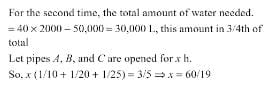QUESTION: 6

Refer to the data below and answer the questions that follow.

Anoop and Sandeep can dig a well each in 10 and 5 days respectively. But on a particularly difficult terrain the work is such that due to fatigue every' subsequent day the efficiency of a worker falls by 10%.
Q.If Anoop is given a task of digging one such well in the difficult terrain, then in how many days will he finish the work?

Solution:

Explanation : The total no. of days in which Anoop can dig the well is 10 days.

Anoop's one day efficiency is 10%.

On day one Anoop performs 10% of his work efficiency, then the next day he won't be able to perform because as per the question the efficiency of a worker falls by 10%.

thus, 10%-10% = 0.

QUESTION: 7

A and B together can complete a work in 3 days. They start together but after 2 days, B left the work. If the work is completed after two more days, B alone could do the work in

Solution:

(A+B)'s one day's work = 1/3 part
(A+B) works 2 days together = 2/3 part
Remaining work = 1−2/3 = 1/3 part

1/3 part of work is completed by A in two days
Hence, one day's work of A = 1/6
Then, one day's work of B = 1/3−1/6 = 1/6
So, B alone can complete the whole work in 6 days.

QUESTION: 8

Refer to the data below and answer the questions that follow.

Anoop was writing the reading comprehension sections in Lhe DOG entrance examinations, There were four passages of exactly equal length in terms of number of words and die four passages had 5, 8, 8 and 6 questions following each of them respectively. It is known that Anoop can answer exactly 12 questions in the time he takes to read any one of the four passages. Assume that his rate of reading and answering questions remains the same throughout the section.

Q.

By what per cent should Anoop increase his reading speed if he has to cut down on his total time spent on the section by 20%? Assume that the time spent on answering the questions is constant and as given in the directions.

Solution: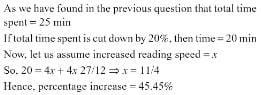QUESTION: 9

A can work half as fast as B and C together. A and B together can work three times as fast as C. If all three of them complete a job in 15 days, how long would C take to complete the same work?

Solution:

Let us assume that

A finishes the work alone in a days

B finishes the work alone in b days

C finishes the work alone in c days

So according to the question,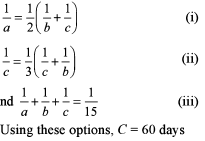QUESTION: 10

In what time would a cistern be filled by three pipes of diameter of 1 cm, 2 cm and 3 cm if the largest pipe alone can fill the cistern in 49 minutes, the amount of water flowing through each pipe being proportional to the square of its diameter?

Solution:

Since the amount of water flowing through each pipe is proportional to square of its diameter so if efficiency of longest pipe (3 cm) = 1/49

Then efficiency of pipe (2 cm) = 4/(49 x 9)

and efficiency of pipe (1 cm) = 1/ (49 x 9)

Now let cistern is filled by all three pipes in x minutes.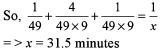QUESTION: 11

Sagar is 20% more efficient than Pranesh. If Pranesh can complete a piece of work in 30 days, then in how many days can both Sagar and Pranesh complete the work?

Solution: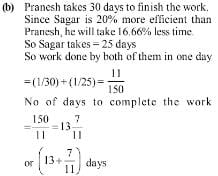QUESTION: 12

A factory manufactures dyes. It has 12 men and two machines which can be operated by all of its men. It takes 4 hours to manufacture one dye on the machine with the operator. The machines can work continuously without a break. Without the machine each of the men can manufacture a dye in 8 hours. The policy is such that the production is maximized and the men are ready to work in three shifts of 8 hours per day. What will be the average cost incurred per dye if 1 man hour costs Rs 20 and 1 machine hour costs Rs 15?

Solution:

To maximize the production, four men will work in each shift. 2 men will work with machines and 2 men work alone.
Total cost incurred in one hour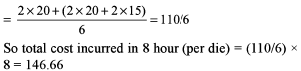QUESTION: 13

A, B, C, D and E are five taps. The capacity of B is 2 times that of A, the capacity of C is 3 times that of A. Capacities of D and E are 4 and 5 times that of A respectively. In the first case A, C and E act as input pipes and B and D act as output pipes.In the second case, C, D, E act as input pipes and A and B act as output pipes.If A and B working together as input pipes can fill the tank in 4 hours, then what is the difference in the time required to fill the tank in the first and second case stated above?

Solution:

Let B take x hours to fill the tank, so according to the question, time required by each,

A = 2x
B=  x
C= 2x/ 3
D= 2x/4
E= 2x/5

A and B together fills the tank in 4 hours.

=> 1/2x +  1/ x = 1 /4

=>  x = 6

In first case, when A, C and E act as input pipes and B and D as output pipes;

Total time = 1/ 12 + 1/ 4 + 5/ 12 -1/ 6 - 1/ 3

=> 4 hours

In 2 nd case, when C, D and E act as input pipes and A and B  as output pipes;

Total Time = ¼ + ⅓ +5/12- 1/12-⅙-⅓

=> 4/3 hours

QUESTION: 14

Anil, Bhuvan and Chandan take 10,20 and 25 days to complete a job. What is the minimum time required to finish the job if not more than 2 of them work together on a single day and no two consecutive days have the same pair of people working?

Solution:

Assume total amount of work =100 units

A does = 10 units/day,

B does = 5 units/day and

C does = 4 units/day

Possible Pairs:

A + B = 15 units/day,

A + C = 14 units/day,

B + C = 9 units/day

To minimize time, we will use the first two pairs.
So, 15 + 14 + 15 + 14 + 15 + 14 + 15 = 102 Units

So, 7 days are required.

QUESTION: 15

Anuj can do a piece of work in a certain number of days. To do the same piece of work, Bhanu takes thrice the number of days as Anuj takes whereas Chandu takes thrice as many days as Bhanu does and Dodo takes thrice as many days as Chandu does. Now, they are paired and two groups are formed. The first pair takes one-third the time taken by the second pair to complete the work, which is the first pair?

Solution:

Let Anuj do the work in x days.

So, Bhanu = 3x days, Chandu = 9x days and Dodo = 27x days

Now, using options,

Anuj + Chandu = 1/x + l/9x = 10/9x,

So, they take 9x/10 days

Bhanu + Dodo = l/3x + 1/27x = 10/27x, So, they take 27x/10 days

Now, 1/3 of 27x/ 10 = 9x /10.

So, Anuj and Chandu is first pair.Use Code STAYHOME200 and get INR 200 additional OFF Use Coupon Code